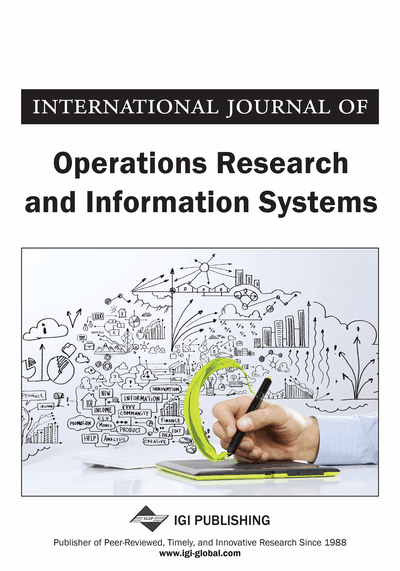# Numerical Solution for a Transient Temperature Distribution on a Finite Domain Due to a Dithering or Rotating Laser Beam

Tsuwei Tan (Department of Applied Mathematics, Naval Postgraduate School, Monterey, CA, USA) and Hong Zhou (Department of Applied Mathematics, Naval Postgraduate School, Monterey, CA, USA)
DOI: 10.4018/ijoris.2013100102
Available
\$37.50
No Current Special Offers

## Abstract

The temperature distribution due to a rotating or dithering Gaussian laser beam on a finite body is obtained numerically. The authors apply various techniques to solve the nonhomogeneous heat equation in different spatial dimensions. The authors’ approach includes the Crank-Nicolson method, the Fast Fourier Transform (FFT) method and the commercial software COMSOL. It is found that the maximum temperature rise decreases as the frequency of the rotating or dithering laser beam increases and the temperature rise induced by a rotating beam is smaller than the one induced by a dithering beam. The authors’ numerical results also provide the asymptotic behavior of the maximum temperature rise as a function of the frequency of a rotating or dithering laser beam.
Article Preview
Top

## 1-D Mathematical Modeling

Consider a laser beam hitting a 1-D rod with finite length. The beam moves along the rod which is insulated at the two endpoints. Mathematically, the temperature distribution of the rod can be modeled by the nonhomogeneous heat equation:

(1) wheredenotes the temperature rise of the rod at positionand time,is the thermal diffusivity of the rod,is the thermal conductivity, andis the energy distribution of the moving laser beam. In the case of a dithering laser beam shown in Figure 1 (1),can be expressed as(2)whereis the position of the dithering Gaussian beam,is the initial position of the laser beam,is the intensity of the laser beam,is the effective radius of the laser beam, andis a constant used for the Gaussian model. The initial condition foris zero which assumes that the rod has the same temperature as the ambient initially. The boundary conditions impose that the rod is insulated at the two ends:

## Complete Article List

Search this Journal:
Reset
Volume 13: 1 Issue (2022): Forthcoming, Available for Pre-Order
Volume 12: 4 Issues (2021)
Volume 11: 4 Issues (2020)
Volume 10: 4 Issues (2019)
Volume 9: 4 Issues (2018)
Volume 8: 4 Issues (2017)
Volume 7: 4 Issues (2016)
Volume 6: 4 Issues (2015)
Volume 5: 4 Issues (2014)
Volume 4: 4 Issues (2013)
Volume 3: 4 Issues (2012)
Volume 2: 4 Issues (2011)
Volume 1: 4 Issues (2010)
View Complete Journal Contents Listing